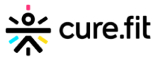New update is available. Click here to update.
Last Updated: 11 Dec, 2020

# Job Scheduling Problem

Moderate+1 more companies

## Problem statement#### You are required to schedule the jobs in such a way that total profit will be maximized. Only one job can be scheduled at a time, and jobs can be scheduled at only integer moments of time greater than or equal to one.

##### For example-
``````Let there be three jobs ‘A’, ‘B’, and ‘C’-
•Profit and deadline associated with job ‘A’ being 20 and 1.
•Profit and deadline associated with job ‘B’ being 30 and 2.
•Profit and deadline associated with job ‘C’ being 40 and 2.

We will perform job ‘C’ at time t = 1 and job ‘B’ at time t = 2. The total profit will be 70. There is no other sequence of jobs which can fetch us a better overall profit.
``````
##### Input Format
``````The first line of input contains an integer ‘T’ denoting the number of test cases to run. Then the test case follows.

The first line of each test case contains a single space-separated integers ‘N’, denoting the total number of jobs.

The next ‘N’ lines contain two space-separated integers, 'deadline[i]' and 'profit[i]' denoting the deadline and the profit associated with the 'i-th' job.
``````
##### Output Format :
``````For each test case, print the maximum profit after scheduling all the jobs in an optimal manner.

Output for each test case will be printed in a new line.
``````
##### Note
``````You do not need to print anything; it has already been taken care of. Just implement the given function.
``````
##### Constraints:
``````1 <= T <= 5
1 <= N <= 10 ^ 3
1 <= profit[i] <= 10 ^ 9

Time Limit: 1 sec
``````## Approaches

### 01 ApproachThe key observation here is that we will always try to maximize our profit by giving priority to the job with maximum profit. Initially, we will sort the jobs according to their profits and then check for each job as to whether it is possible to perform it.

The algorithm will be-

• We will initialize an array/list ‘time’ of length N, with each time[i] denoting whether a job is scheduled at the i-th moment.
• We will sort the jobs according to their profits.
• For each job i, we will:
• We will begin iterating from deadline[i] to 1 and find the maximum time where no job is scheduled by querying the time array.
• If we get the required moment, ‘optTime’:
• Set time[optTime] to 1.
• Increase our final answer by profit[i].
• Else, we skip the current job.